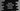# Python map(lambda) function explanation with example## Python map(lambda) function example :

map() is used to apply one function to each element of an iterable like list, tuple in python. map() takes one function as its argument and uses that function on each element of the iterable. It returns the modified iterable.

### A simple example of map():

map takes one function and one iterable as its parameters. It applies that function to each element of the iterable. This method is defined as below :

``map(func, iterable)``

Let’s take a look at the example below :

``````def square(x):
return x*x

numbers = [1, 2, 3, 4, 5]

square_numbers = list(map(square, numbers))

print(square_numbers)``````

The return value of this function is an object. We need to convert it to a list using a list constructor.

It will print :

``[1, 4, 9, 16, 25]``

### map() with lambda :

We can also pass one lambda instead of a function to map :

``````numbers = [1, 2, 3, 4, 5]

square_numbers = list(map(lambda x: x*x, numbers))

print(square_numbers)``````

This will give the same result.

``[1, 4, 9, 16, 25]``

### map() with two lists :

map() can accept more than one iterables:

``````numbers_list_1 = [1, 2, 3, 4, 5]
numbers_list_2 = [6, 7, 8, 9, 10]

square_numbers = list(map(lambda x,y: x+y, numbers_list_1, numbers_list_2))

print(square_numbers)``````

We are passing two lists and the lambda adds the values of both lists. It will print the below output :

``[7, 9, 11, 13, 15]``